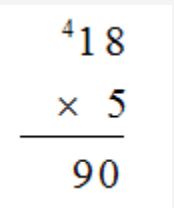### Does the curriculum require that students use multiple strategies to solve the same problem? 1

Why do recommended resources (e.g., textbooks) show multiple strategies for the same math problem?

Resources such as textbooks, websites and computer applications can all be used to support student achievement of curricular outcomes. The recommended resources in Saskatchewan are also used in other provinces and therefore were created to serve a wide audience. Textbooks must meet the needs of classroom teachers, students, tutors and home schooling teachers. As a result, textbooks contain more information, solution strategies or detailed calculation explanations than classroom teachers may need.

Does the curriculum require that students use multiple strategies to solve the same problem?

Saskatchewan curricula require that students, “develop strategies for, and carry out multiplication of whole numbers” (outcome N5.2). Students should use strategies that they have come to understand. Students solving the same problem may choose different strategies. The math curriculum does not require that students solve a problem by demonstrating multiple strategies. Instead, students should work toward choosing a strategy that is efficient for a given question.

A class discussion of how everyone solved the problem allows students to listen and to make sense of other students’ solutions in order to build conceptual understanding. Students will then be able to draw from a range of strategies as they attempt to solve new problems.

#### Strategy

If students are asked to multiply 18 x 5, the standard algorithm is one acceptable strategy. However, some students may solve the same problem by using a ‘double and halve’ strategy and instead multiply 9 x 10. Flexibility with numbers leads students to recognize that 5 doubles to 10, which is a helpful strategy for students because 10 is an easier number to multiply. Conceptual understanding of multiplication dictates that when a student doubles one number, they must halve the other one (in this case half of 18).

Standard Algorithm18 x 5

Halve and Double

9 x 10 = 90

When students are asked, for example, to subtract 2354-999, the standard algorithm strategy involves a sequence of borrowing. If students understand more than the standard algorithm they may choose to change the question to 2354-1000. Changing 999 to 1000 eliminates the borrowing sequence which is easier to calculate. As students subtracted 1 more than needed, they have to add one to the final answer.

Standard Algorithm“Friendly” numbers

2354 – 1000 = 1354

I subtracted one more than needed so